# An Introduction to Plotting

import pandas as pd

import seaborn as sns
%matplotlib inline

### Single Variable: Continuous

#### Histogram

When you have a single continuous variable and want to visualise the distribution of its values in your dataset, a histogram is generally what you need. This groups the values into bins, where each bin is an interval within the range of values your variable can take. The x axis will show the interval of each bin, while the y axis shows the number of values in your dataset that fall within that interval.

Let's load in some data using seaborn's handy `load_dataset()` function. The `flights` dataset has three variables: two ordered categorical (`year``month`) and one continuous (`number of passengers`).

Input:

Output: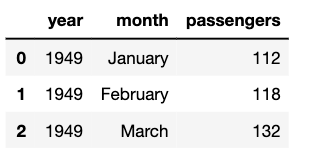A simple histogram will show the overall distribution of the `passenger` variable. This is easy to plot, as pandas dataframes have a built-in method for generating it.

Input:

import pandas as pd

import seaborn as sns
%matplotlib inline

Output: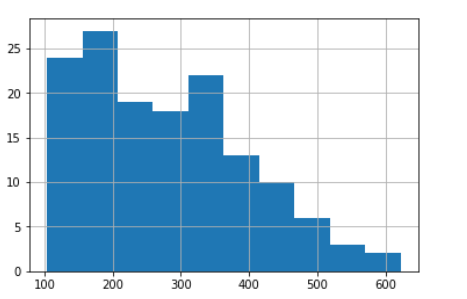By default, pandas plots histograms using 10 bins but you could fine-tune this. Displaying more bins gives a more detailed overview of the distribution, up to a point: it all depends on how many observations you have overall and how they are distributed. You can see how using 20 bins shows more information about the distributions inside the larger 5 bins.

Input:

flights.passengers.hist(bins=5) # The blue bars
flights.passengers.hist(bins=20) # The orange bars

` `

Output: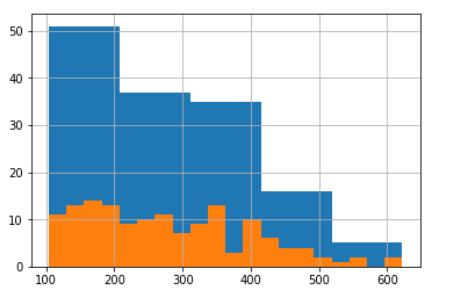So the range of passenger numbers is a little over 100 to a bit over 600, with most flights towards the lower end. For a more precise overview, the `describe`method for a dataframe's columns will give general descriptive statistics.

Input:

`flights.passengers.describe()`

Output:

`count     144.000000 mean      280.298611 std       119.966317 min       104.000000 25%       180.000000 50%       265.500000 75%       360.500000 max       622.000000 Name: passengers, dtype: float64`

### Boxplot

For a visual representation of `describe`, a boxplot will show the minimum and maximum values (the left and right whiskers), the range of values covered by the 25th to 75th percentiles (the box) and the value of the median (the line inside the box).

Input:

`sns.boxplot(x=flights.passengers)`

Output: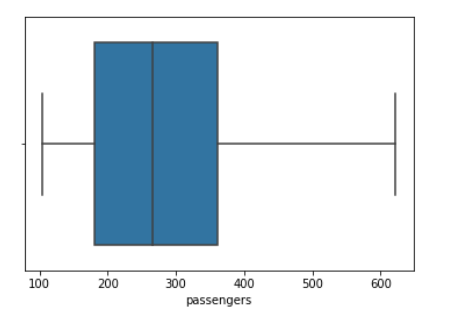## Single Variable: Categorical

### Bar chart

When you have a variable which takes on named, rather than numerical, values then the most common way of representing them is with a bar chart.

Here, we'll load the `titanic` dataset. Each row is a passenger on the ship, while the `class` variable gives the class of that passenger's ticket.

titanic['class'].value_counts()

` `

Output:

Third 491

First 216

Second 184

Name: class, dtype: int64

` `

You can chain `.plot(kind='bar')` to the above `value_counts()` method, but I prefer to use seaborn as you can directly pass it the original data. It will then do the counting for you and allow you more control over appearance. For example, if you do not like the ordering seaborn used for the x axis, then you can set it manually as a list e.g. `order=['Third', 'Second', 'First']`

Input:

sns.countplot(titanic['class'])

` `

Output: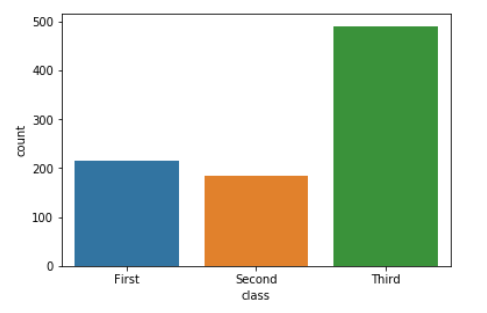If you want to normalise the counts so as to see relative percentages rather than counts, then you just need to do that to the data before plotting it as a normal barplot.

Input:

titanic_normed = pd.DataFrame(titanic['class'].value_counts(normalize=True)).reset_index() sns.barplot(data=titanic_normed, x='index', y='class')

` `

Output:## Plotting relationships between variables

Above, we only had a single variable. We examined it by looking at the frequency of values (the histogram) or by plotting descriptive statistics (the boxplot). But often we want to see how one variable is linked to another - as the value of one variable changes, what happens to the value of the other variable?

With continuous and ordered/unordered categorical variables, we have four possible combinations. Let's look at them in turn.

## Continuous x continuous

The `mpg` dataset contains information about cars, measuring their weight, fuel efficiency and so on. We might expect heavier cars to have lower fuel efficiency.

When plotting continuous variables, the one you place on the x-axis should be the independent variable. This is generally some property or value we observe. The y-axis should display the dependent variable. This is a function of the values on the x-axis and is generally something we measure for each observed value on the x-axis. Here, we will place weight on the x-axis and miles per gallon on the y-axis.

Generally, the best choice of visualisation for this is a scatterplot. Each point represents the relation between a single value on the x-axis and its corresponding y value.

Input:

g = sns.scatterplot(data=mpg, x='weight', y='mpg')

` `

Output:There are several variations on this, which are made available through seaborn's `jointplot`. The default will add histograms on the margins, for each of the two variables.

Input:

` `

Output: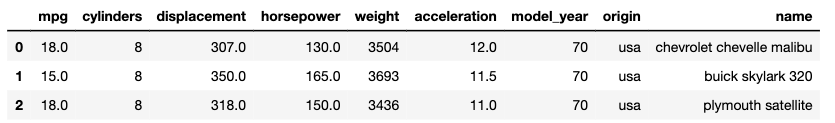Input:

sns.jointplot(data=mpg, x='weight', y='mpg')

` `

Output: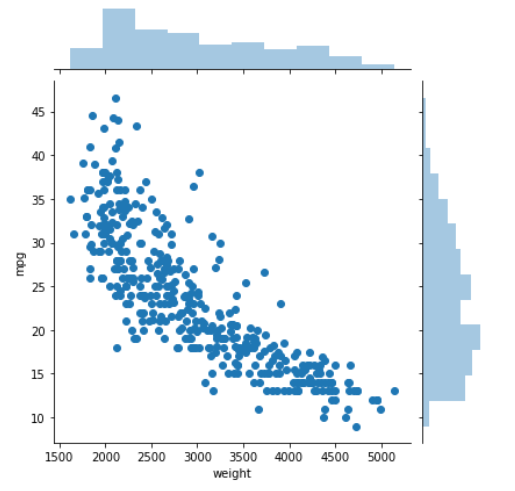By setting the `kind` argument to `kde`, you can instead plot a joint kernel density estimate, with individual density estimates on the margins.

Input:

sns.jointplot(data=mpg, x='weight', y='mpg', kind='kde')

` `

Output: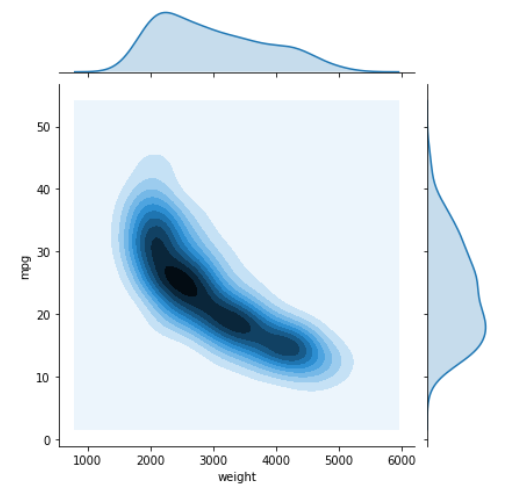Or you can set it to `hex` and plot the values as hexagons, which represent histogram-type bins. This can be very useful if you have a lot of observations in your dataset and plotting all those points is slow or messy.

Input:

sns.jointplot(data=mpg, x='weight', y='mpg', kind='hex')

Output: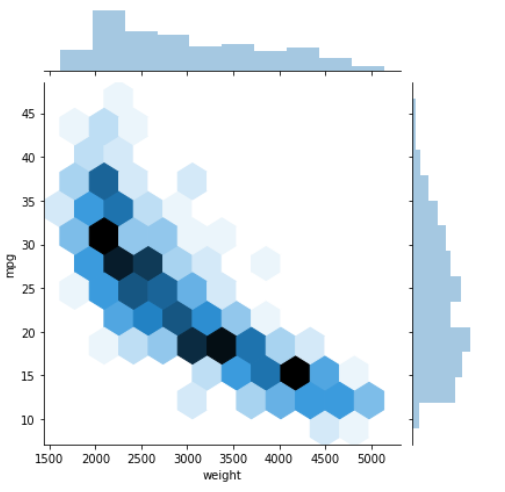## Continuous x unordered categorical

There are a few more options when it comes to jointly plotting continuous and categorical data. In general, the categorical data will go on the x-axis and you may need to change the order in which they are displayed.

Let's look at the relationship between fuel efficiency (continuous) and a car's country of origin (unordered categorical). Seaborn's will`stripplot` make a separate scatterplot for each categorical variable and place it on the x axis, with its own colour. It will also stagger the points a little to help see their distribution - this can be controlled with the `jitter` argument.

Input:

sns.stripplot(data=mpg, x='origin', y='mpg', jitter=0.3)

` `

Output: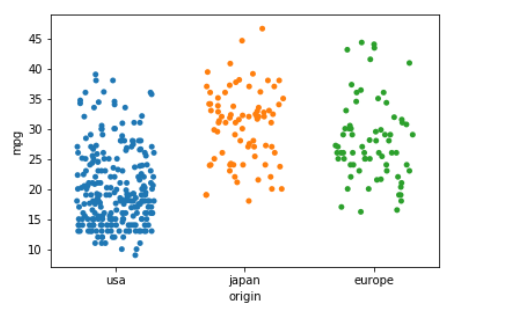The `swarmplot` does the same but arranges the points so that there is no overlapping.

Input:

sns.swarmplot(data=mpg, x='origin', y='mpg')

` `

Output: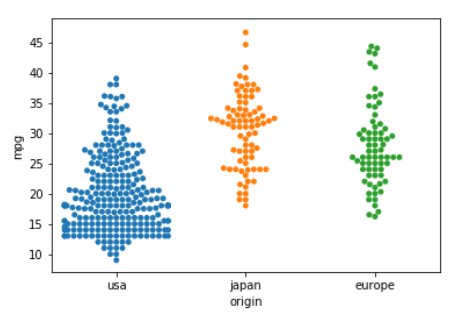And if you want a boxplot for each categorical variable, there is no need to do them separately and manually place them in a figure - `catplot` is a great way to plot categorical x continuous data.

Input:

sns.catplot(data=mpg, x='origin', y='mpg', kind='box')

` `

Output:## Continuous x ordered categorical

Sometimes, the categorical data will have a natural order to it. The most common of these is times or dates. This can sensibly be plotted as a line, to show how the continuous variable changes over time. Generally, the categorical data must be unique - no value should appear more than once.

The `gammas` dataset contains fMRI measurements taken from multiple subjects. Let's look at subject 0, and see how a signal which is dependent on blood oxygen levels (BOLD signal) changed over time in various regions of interest (ROI) in the brain.

Seaborn's `lineplot` method has a `hue` argument, that will seperate out the three different values for ROI and plot them as their own lines.

Input:

subject_0_data = gammas[(gammas.subject == 0)]

sns.lineplot(data=subject_0_data, x='timepoint', y='BOLD signal', hue='ROI')

` `

We could also focus on a particular ROI and then see how all subjects compare by setting `hue="subject"`

Input:

sns.lineplot(data=gammas[gammas.ROI == 'IPS'], x='timepoint', y='BOLD signal', hue='subject', legend=False)
# Remove the legend as it gets in the way with the default plot size.

` `

Output:## Categorical x categorical

The most common non-graphical way of representing two joint categorical variables is as a contingency table. Each row of the table represents a possible value of one variable, the columns of the other variable. Cells are populated with the number of observations of pairs of those values.

We can create that table using pandas' `crosstab` function - just tell it which columns of a dataframe to use.

Input:

sex_class = pd.crosstab(titanic.sex, titanic['class'])

sex_class

` `

Output:We can also normalise the values to show percentages, rather than counts.

Input:

sex_class_normed = pd.crosstab(titanic.sex, titanic['class'], normalize=True) * 100
sex_class_normed

` `

Output:This tabular data is easily to represent visually as a heatmap. This essentially colours in the cells of the table, based on their value. It can be a great way to very quickly communicate the joint distribution of two categorical variables, especially where you want to highlight the fact that some particular combinations are very high or low.

Input:

sns.heatmap(sex_class, cmap='Blues', square=True, annot=True, fmt='g')

Output:Input:

sns.heatmap(sex_class_normed, cmap='Blues', square=True, annot=True, fmt='.2f', cbar=False)

Output:# Summary

## Questions to ask before plotting

Here are the questions to ask before you start plotting:

• What is the purpose of my visualisation?

• Show the relationship between variables?
• Illustrate individual distributions of variables?
• What kind of variables do I have? For each variable:

• Is it continuous?
• Or is it categorical?
• Besides these variables, is there some other informative distinction I want to show? Do my variables come from...

• different groups of people/individuals/companies/locations?
• different time periods?
• different experiments?
• different models?
• Have I included all the necessary information?

• Descriptive title?
• Informative caption?
• Axes have suitable labels?
• Units for axes, where appropriate?
• Axes using suitable scale?
• Do I need a legend?
• Do my colours and styling aid readability?

## Cheat sheet: picking a visualisation for your data

And a quick list, linking types of data to types of visualisation:

### Single variable

• continuous
• histogram: more visual, big picture, show distribution of ranges of values
• boxplot: more statistical and detailed
• categorical
• barchart: show counts or proportions of values

### Joint variables

• continuous x continuous

• scatterplot: show relation between every x and y
• basic jointplot: as above, but with marginal histograms per variable
• kde jointplot: show distribution of joint values, with individual histograms
• hex jointplot: as above, but points are now mini-histograms
• continuous x unordered categorical

• stripplot: multiple scatterplots arranged on x axis
• swarmplot: as above, but no overlapping points allowed
• catplot with boxplots: replace individual plots with boxplots
• continuous x ordered categorical
• line: shows exactly what values are seen over time
• categorical x categorical
• cross-tabulate then heatmap: show relative proportions of joint variables

# Next steps

• Look into seaborn's documentation for figure aesthetics and choosing colour palettes - these can make your visualisations look really great. The ones I did here use the default settings and could definitely be improved upon!

• Think about how the plots could be improved in terms of the questions under "Have I included all the necessary information?". Seaborn makes it very easy to add titles and so on to figures.

• Seaborn also makes it easy to visualise many aspects of the data at once, rather than individually as we did here. Read the documentation for jointplot and catplot to see how flexible and easy to use these methods are!

• Try applying the above to real data that you have, rather than the toy datasets used here.Alexander Robertson is a Data Science PhD student at the University of Edinburgh, where his research focuses on variation, usage and change in natural language and also emoji.## Enquire now

Fill out the following form and we’ll contact you within one business day to discuss and answer any questions you have about the programme. We look forward to speaking with you.